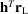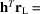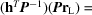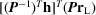International
Tables for
Crystallography
Volume B
Reciprocal space
Edited by U. Shmueli

International Tables for Crystallography (2010). Vol. B, ch. 1.4, pp. 114-115   | 1 | 2 |

## Section 1.4.2.1. Point-group symmetry of the reciprocal lattice

U. Shmuelia

#### 1.4.2.1. Point-group symmetry of the reciprocal lattice

| top | pdf |

Regarding the reciprocal lattice as a collection of points generated from a given direct lattice, it is fairly easy to see that each of the two associated lattices must have the same point-group symmetry. The set of all the rotations that bring the direct lattice into self-coincidence can be thought of as interchanging equivalent families of lattice planes in all the permissible manners. A family of lattice planes in the direct lattice is characterized by a common normal and a certain interplanar distance, and these two characteristics uniquely define the direction and magnitude, respectively, of a vector in the reciprocal lattice, as well as the lattice line associated with this vector and passing through the origin. It follows that any symmetry operation on the direct lattice must also bring the reciprocal lattice into self-coincidence, i.e. it must also be a symmetry operation on the reciprocal lattice. The roles of direct and reciprocal lattices in the above argument can of course be interchanged without affecting the conclusion.

The above elementary considerations recall that for any point group (not necessarily the full point group of a lattice), the operations which leave the lattice unchanged must also leave unchanged its associated reciprocal. This equivalence of point-group symmetries of the associated direct and reciprocal lattices is fundamental to crystallographic symmetry in reciprocal space, in both points of view mentioned in Section 1.4.1.

With regard to the effect of any given point-group operation on each of the two associated lattices, we recall that:

 (i) If P is a point-group rotation operator acting on the direct lattice (e.g. by rotation through the angle α about a given axis), the effect of this rotation on the associated reciprocal lattice is that of applying the inverse rotation operator,(i.e. rotation throughabout a direction parallel to the direct axis); this is readily found from the requirement that the scalar product, where h andare vectors in the reciprocal and direct lattices, respectively, remains invariant under the application of a point-group operation to the crystal. (ii) If our matrix representation of the rotation operator is such that the point-group operation is applied to the direct-lattice (column) vector by premultiplying it with the matrix P, the corresponding operation on the reciprocal lattice is applied by postmultiplying the (row) vectorwith the point-group rotation matrix. We can thus write, e.g.,. Note, however, that the orthogonality relationship:is not satisfied if P is referred to some oblique crystal systems, higher than the orthorhombic.

Detailed descriptions of the 32 crystallographic point groups are presented in the crystallographic and other literature; their complete tabulation is given in Part 10of Volume A (IT A, 2005).

### References

International Tables for Crystallography (2005). Vol. A, Space-Group Symmetry, edited by Th. Hahn, 5th ed., corrected reprint. Heidelberg: Springer.